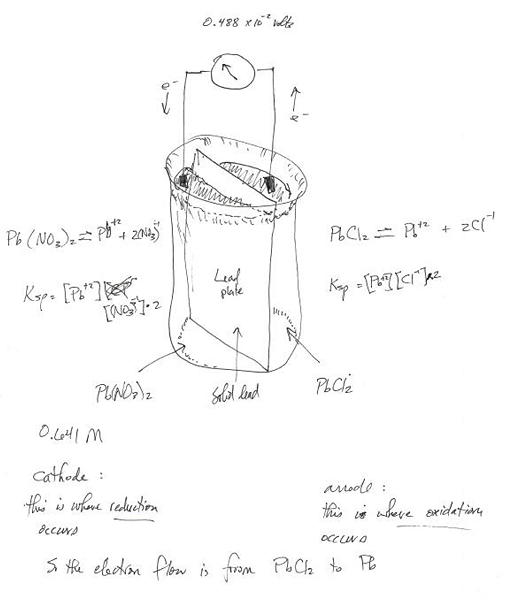Select Page

# Ksp

by | Apr 11, 2021

Ksp Calculation using the Nernst Equation

\begin{equation} \begin{split} {E}_{cell} & = {E}^{\circ }-\frac{RT}{nF}\times ln(Q) \\ ln(Q) & = ({E}_{cell} - {E}^{\circ })\times \left(-\frac{nF}{RT}\right) \\ &=\frac{x\ moles\ {PbCl}_{2}}{0.693\ moles\ {{Pb(NO}_{3})}^{2}} \\ \\ &; where\ Q= the\ reaction\ quotient \\ &;and\ {E}_{cell}=the\ voltage\ across\ the\ lead\ plate \\ &;and\ R = molar\ gas\ constant,\ \approx 8.314\ Joules/mole\ K \\ &;and\ F=Faraday\ constant,\ \approx 96,485 \\ &;\ \ \ \ \ \ \ \ \ \ \ \ (\sim 9.65\times {10}^{4})\ Coulombs/mole\ K \\ \end{split} \end{equation}
Example Problem

A student does an experiment to determine the molar solubility of lead(II) chloride. He constructs a voltaic cell at 298 K consisting of 0.693 M lead nitrate solution and a lead electrode in the cathode department, and a saturated lead chloride solution and a lead electrode in the anode compartment.
If the cell potential is measure to be 4.96 X 10^-2 V, what is the value of {K}_{sp} for lead chloride at 298 K based on this experiment?

Once again, here are the givens.
\begin{equation} \begin{split} {E}_{cell} & = 0.0496\ or\ {4.96}^{-2}\ Volts \\ n & = 2\ moles\ of\ electrons\ transferred \\ & \ \ \ \ \{i.e.,\ {Pb}^{\circ }+2{Cl}^{\circ }\rightleftharpoons {PbCl}_{2}+2{e}^{-}\} \\ {E}^{\circ }&=0.0000\ Volts \\ & \ \ \ \ \{the\ voltage\ at\ steady\ state\ equilibrium\} \\ T & =298\ K \\ & \ \ \ \ \{temperature\ in\ Kelvin\ degrees\} \end{split} \end{equation}
Calculating the Result
\begin{equation} \begin{split} 0.0496\ V & = 0.0000\ V -\frac{8.314\ Joules/mol\ K\times 298\ K}{2\ moles\ {e}^{-}\times 96,485\ Coulombs/mole}\times ln\left(\frac{\ M\ moles\ {PbCl}_{2}}{0.693\ moles\ {{Pb(NO}_{3})}^{2}}\right) \\ ln\ \left(\frac{x\ M}{0.693}\right)&=(0.0496-0.0000)\times\left(- \frac{2\times 96,500}{8.314\times 298}\right) \\ ln\ \left(\frac{x\ M}{0.693}\right)&=\ \sim -3.864 \\ x\ M&=\ \sim 0.693\times {e}^{-3.864} \\ &=\ \sim 0.693\times 2.10\times {10}^{-2} \\ x\ M&=\ \sim 1.46\times {10}^{-2}\ molar\ solubility\ of\ {PbCl}_{2} \\ \end{split} \end{equation}
Ksp Calculation for a Saturated Lead Chloride Solution
In the equation.
\begin{equation} \begin{split} {PbCl}_{2}&\rightleftharpoons {Pb}^{-2}+2{Cl}^{-1} \\ [{PbCl}_{2}]&=x\ M \\ [{Pb}^{2+}]&=x\ M \\ [{Cl}^{-}]&=2x\ M \\ \\ \end{split} \end{equation}
So plugging this all in for the solubility product
\begin{equation} \begin{split} {K}_{sp}&=[{Pb}^{2+}]\times {[{Cl}^{-}]}^{2} \\ &=(x)\times {(2x)}^{2} \\ &=x\times 4\times {x}^{2} \\ &=4\times {x}^{3} \\ &=4\times {(1.46\times {10}^{-2})}^{3} \\ {K}_{sp}&=\ \sim 1.2\times {10}^{-5} \\ \end{split} \end{equation}

## Discussion

Nernst equation describes the way charges can cross a membrane, or in the problem above, a metal plate separating two solutions. The voltage across a membrane or a plat is related to the number of electrons transferred.

The here is that the solution of the PbCl2 is saturated. With the metal plate the saturation begins changing immediately whn you put the solutions in contact with the plate on their respective sides. However, with a cell membrane there are actually ion pumps and gates that come into play. This is important in understanding how every nerve, muscle, and cardiac cell performs its physiological task. The question ins’t practicable when I treat patients, but the understanding of the principle is.

Notice above that the solubility product Ksp is not the same as solubility Ks.